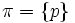# P-core-free group

The article defines a property of groups, where the definition may be in terms of a particular prime that serves as parameter
View other prime-parametrized group properties | View other group properties

## Definition

Let$G$ be a finite group and$p$ be a prime number. We say that$G$ is$p$-core-free if it satisfies the following equivalent conditions:

• The$p$-core (i.e., the largest normal$p$-subgroup of$G$) is trivial. The$p$-core is also termed the Sylow-core, and is the normal core of any Sylow subgroup. It also equals the pi-core where$\pi = \{ p \}$.
•$G$ has no nontrivial normal$p$-subgroup.
•$G$ possesses a faithful irreducible representation over a field of characteristic$p$.

### Equivalence of definitions

The first two definitions are clearly equivalent. For equivalence with the third definition, refer p-core-free iff there exists a faithful irreducible representation over a field of characteristic p.